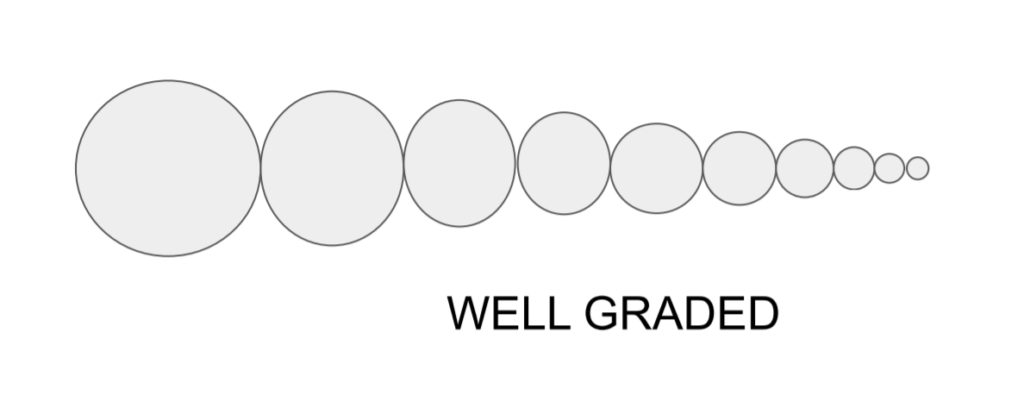# Particle Size Distribution Curve | Properties of Soil | Soil Mechanics

Contents

## Particle Size Distribution Curve

• The result of the particle size distribution analysis is reported in the form of a curve termed as particle size distribution curve, in which % finer is expressed on the Y-axis &size of a particle is expressed on X-axis on a log scale.
• This curve helps in analyzing the type of soil & gradation of soil.
• The soil may be termed well-graded soil. If it has a good representation of all sizes of particle in it.• It soil consist of excess of one size particle & deficiency of another size of particle ( or it consist  of the particle of same size ) it is termed poorly graded soil (in later case it is termed as Uniformly Graded Soil).• It certain size of particle are missing from soil it is termed as gap/flat graded soil.### Particle Size Distribution CurveFor Coarse Grained soil D10, D30, and D60 size of particle holds higher significance & can be found using the particle size distribution curve.

• D10= Size in (mm) such that 10% of particles are finer than this size. It is also termed as efficiency size or effective diameter as defined “By Allen Hagen “.
• D30= Size in (mm) such that 60% of particles are finer than this size.
• D60= Size in (mm) such that 60% of particles are finer than this size.

Their significance is more as this size helps in finding the gradation of Coarse-Grained soil in terms of the following parameters.

### Coefficient of Uniformly (Cu)

It represents particle size range of distribution curve.

It is defined as ratio of D60 size of particles to the D10 size of particles.

$$Cc=\frac{D_{60}}{D_{10}}$$

For uniformly graded soil, Cu, = 1 (or less than 2)

For well graded sand, Cu, > 6.

For well graded Gravel, Cu, > 4

### Coefficient of Curvature (Cc)

If represents shape of particle size distribution curve.

It is defined as

$$Cc=\frac{D_{30}^{2}}{D_{60}·D_{10}}$$

For well-graded soil, Cc should be in the range of 1 to 3

If Cc, is less than 1 or greater than 3, then the soil is gap-graded soil.

 Subject Soil Mechanics Unit Soil Formation & Properties of Soil Topic Particle Size Distribution Curve Next Topic Consistency of Soil Previous Topic Particle Size Distribution

error: Content is protected !!
Scroll to Top